Courses

# Chemical Bonding and Mole Concept

## 25 Questions MCQ Test | Chemical Bonding and Mole Concept

Description
This mock test of Chemical Bonding and Mole Concept for helps you for every entrance exam. This contains 25 Multiple Choice Questions for Chemical Bonding and Mole Concept (mcq) to study with solutions a complete question bank. The solved questions answers in this Chemical Bonding and Mole Concept quiz give you a good mix of easy questions and tough questions. students definitely take this Chemical Bonding and Mole Concept exercise for a better result in the exam. You can find other Chemical Bonding and Mole Concept extra questions, long questions & short questions for on EduRev as well by searching above.
QUESTION: 1

Solution:
QUESTION: 2

Solution:
QUESTION: 3

### .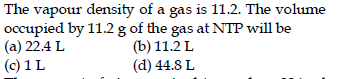Solution:
QUESTION: 4
.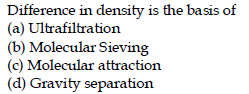Solution:
QUESTION: 5
.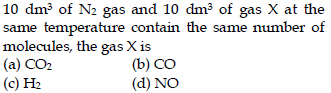Solution:
QUESTION: 6
.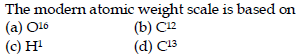Solution:
QUESTION: 7
.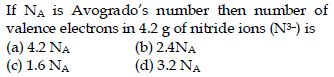Solution:
QUESTION: 8

In allene (C3H4), the type(s) of hybridisation of the carbon atoms is (are)

Solution:
QUESTION: 9
.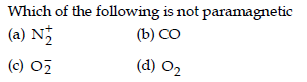Solution:
QUESTION: 10
.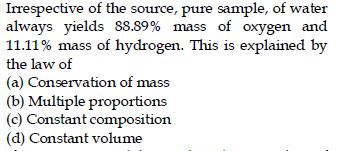Solution:
QUESTION: 11
.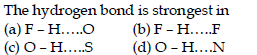Solution:
QUESTION: 12
.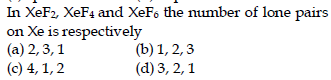Solution:
QUESTION: 13
.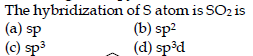Solution:
QUESTION: 14
.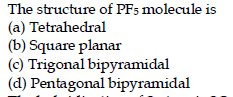Solution:
QUESTION: 15
.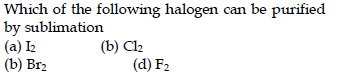Solution:
QUESTION: 16
.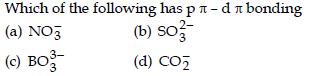Solution:
QUESTION: 17
.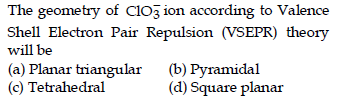Solution:
QUESTION: 18
.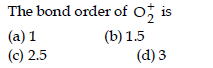Solution:
QUESTION: 19
.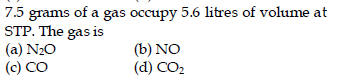Solution:
QUESTION: 20
.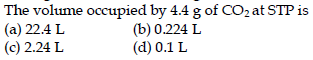Solution:
QUESTION: 21
.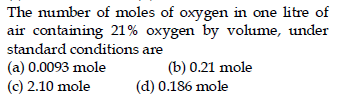Solution:
QUESTION: 22
.Solution:
QUESTION: 23
.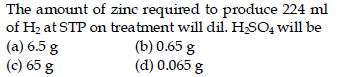Solution:
QUESTION: 24
.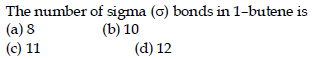Solution:
QUESTION: 25
.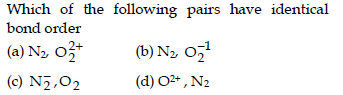Solution: